## XOR Function

The XOR function is a premade function in Google Sheets, which returns TRUE or FALSE based on two or more conditions.

Note: The XOR function is often used together with the IF function.

It is typed `=XOR`:

=XOR(logical_expression1, [logical2_expression, ...])

The conditions are referred to as `[logical_expression1]`, `[logical_expression2]`, and so on.

The conditions can check things like:

• If a number is greater than another number `>`
• If a number is smaller than another number `<`
• If a number or text is equal to something `=`

For two conditions, you can think of the XOR function as: "either this or that, but not both"

## Example XOR Function

Check if the Pokemon type is either Fire type OR has HP less than 60, but not both:

The function returns "TRUE" or "FALSE".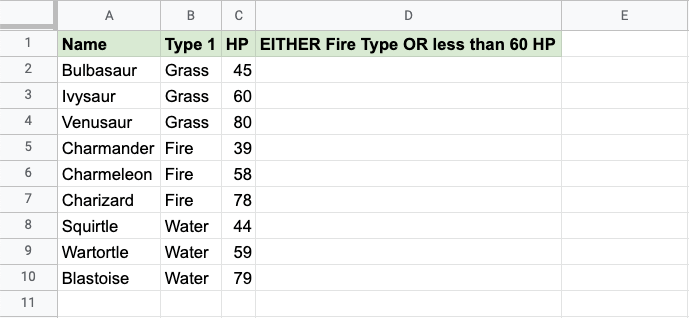Copy Values

Example XOR function, step by step:

1. Select the cell `D2`
2. Type `=XOR`
3. Click the XOR command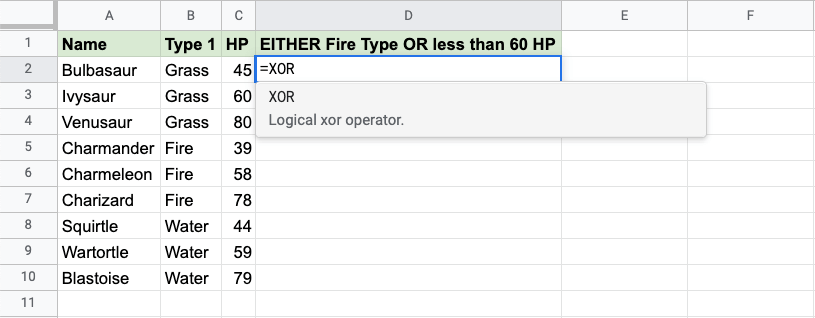1. Specify the first condition `B2="Fire"`
2. Type `,`
3. Specify the second condition `C2<60`
4. Hit enter

Note: You can add more conditions by repeating steps 5-6 before hitting enter.

The XOR function returns "TRUE" if an odd number (1,3,5,..) of conditions are TRUE.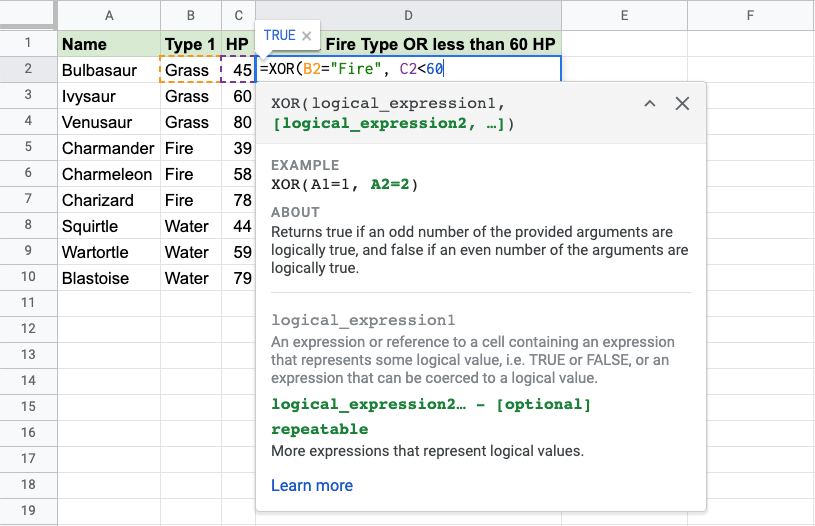Since the value in cell `B2` is not "Fire" the first condition is FALSE.

Since the value in cell `C2` is less than 60 the second condition is also FALSE.

For two conditions, only one condition should to be TRUE for the XOR function to return TRUE.

Note: Text values needs to be in quotes: " "

The function can be repeated with the filling function for each row to perform the same check for each Pokemon: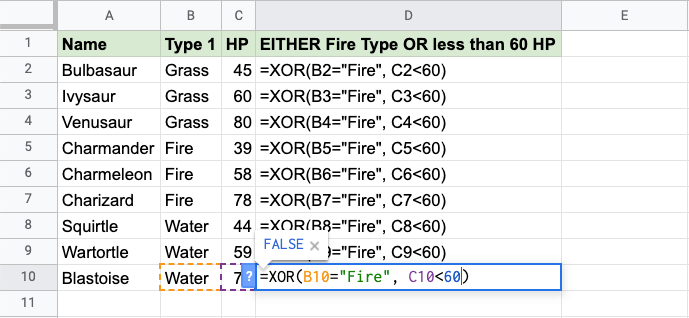Now, each row has a check for Fire Type or HP less than 60, but not both: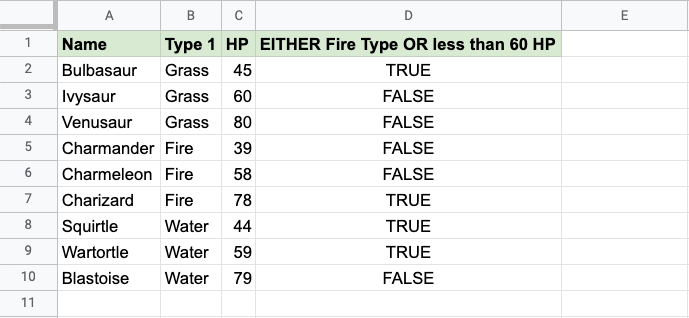For example:

• Ivysaur is not Fire type, but it also does not have less than 60 defense, so the function returns "FALSE".
• Charmeleon is Fire type, and has less than 60 defense, so the function returns "FALSE"
• Wartortle is not Fire type, but has less than 60 defense, so the function returns "TRUE"

## Example XOR Function (with IF)

Combining the XOR function with an IF function lets you check multiple conditions for the IF function:

Note: The IF function lets you specify the return values.

The IF function is typed `=IF` and has 3 parts:

=IF(logical_expression, value_if_true, value_if_false)

The XOR function takes the place of the `logical_expression` condition.

Here, the IF function returns "Yes" or "No".

Example XOR function as the condition for IF function, step by step:

1. Select cell `C2`
2. Type `=IF`
3. Click the IF command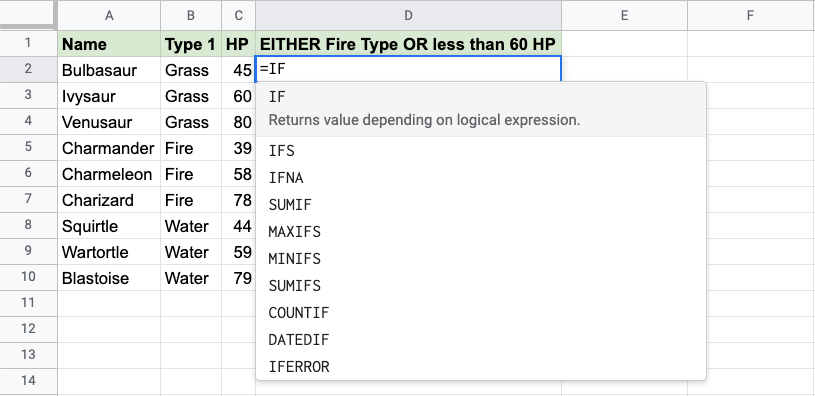1. Type `XOR`
2. Click the XOR command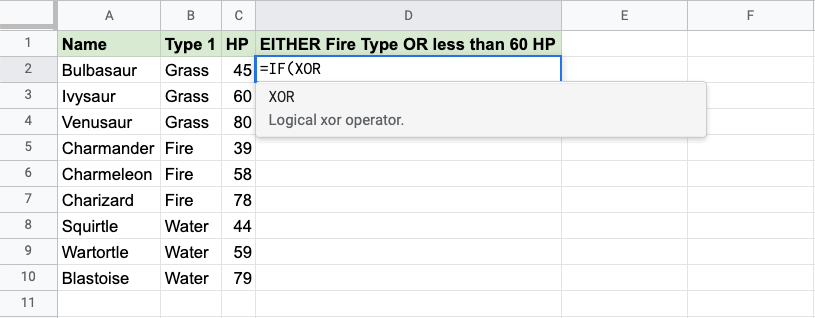1. Specify the first condition `B2="Fire"`
2. Type `,`
3. Specify the second condition `C2<60`
4. Type `),`
5. Specify the value `"Yes"` for when just one condition is TRUE
6. Type `,`
7. Specify the value `"No"` for when both are FALSE, or TRUE at the same time
8. Type `)` and hit enter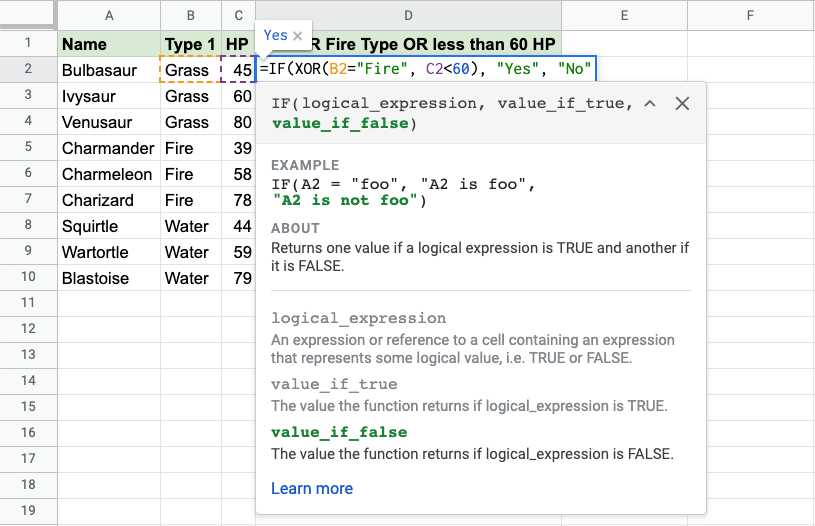The function can be repeated with the filling function for each row to perform the same check for each Pokemon: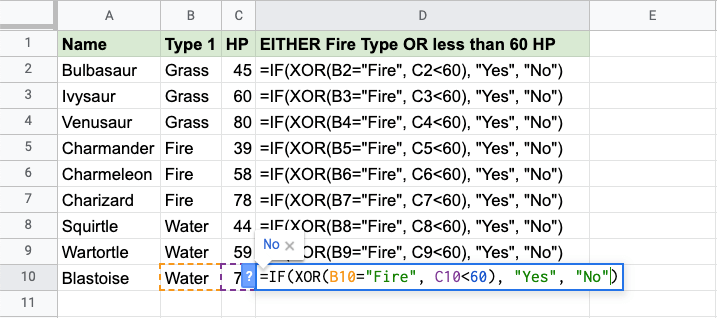Now, each row has a check for Water Type or Defense greater than 60, but not both: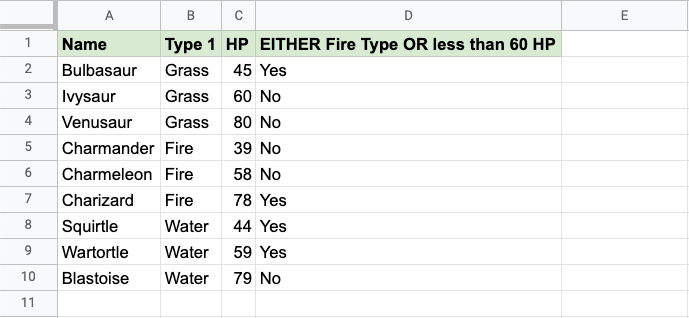For example:

• Ivysaur is not Fire type, but it also does not have less than 60 defense, so the function returns "No".
• Charmeleon is Fire type, and has less than 60 defense, so the function returns "No"
• Wartortle is not Fire type, but has less than 60 defense, so the function returns "Yes"

W3Schools is optimized for learning and training. Examples might be simplified to improve reading and learning. Tutorials, references, and examples are constantly reviewed to avoid errors, but we cannot warrant full correctness of all content. While using W3Schools, you agree to have read and accepted our terms of use, cookie and privacy policy.